Request a Free Counselling Session from our Expert Mentor# NCERT Solution for Class Xth Science Chapter 2 Acid,Base and Salts

Welcome to the NCERT Solution for Class Xth Science Chapter 2 “Acid, Base and Salts” by Swastik Classes. This chapter is an essential part of the Class X Science syllabus, and it deals with the fundamental concepts of acids, bases and salts. The chapter explains the basic properties of acids and bases, and how they react with each other to form salts. It also highlights the different methods of preparation of salts, and their applications in everyday life.
Through the NCERT Solution for Class Xth Science Chapter 2 Acid, Base and Salts by Swastik Classes, you will be able to gain a comprehensive understanding of the concepts of pH scale, indicators and neutralization reactions. You will also learn about the different types of salts, their properties, and how they are used in various industries.
The Swastik Classes provide in-depth explanations and examples to help you understand the chapter better. With the help of these solutions, you will be able to tackle any question related to acids, bases, and salts with ease. By the end of this chapter, you will be equipped with the knowledge to understand and appreciate the role of acids, bases and salts in the world around us.

## Answers of Science NCERT solutions for class 10 Chapter 2 Acid,Base and Salts

Chapter-2

Acid, Base and Salts

Question 1:

You have been provided with three test tubes. One of them contains distilled water and the other two contain an acidic solution and a basic solution, respectively. If you are given only red litmus paper, how will you identify the contents of each test tube?

If the colour of red litmus paper gets changed to blue, then it is a base and if there is no colour change, then it is either acidic or neutral. Thus, basic solution can be easily identified.

Let us mark the three test tubes as A, B, and C. A drop of the solution in A is put on the red litmus paper. Same is repeated with solution B and C. If either of them changes colour to blue, then it is basic. Therefore, out of three, one is eliminated. Out of the remaining two, any one can be acidic or neutral. Now a drop of basic solution is mixed with a drop of each of the remaining two solutions separately and then the nature of the drops of the mixtures is checked. If the colour of red litmus turns blue, then the second solution is neutral and if there is no change in colour, then the second solution is acidic. This is because acidic and basic solutions neutralize each other. Hence, we can distinguish between the three types of solutions.

Question 1:

Why should curd and sour substances not be kept in brass and copper vessels?

Curd and other sour substances contain acids. Therefore, when they are

kept in brass and copper vessels, the metal reacts with the acid to liberate hydrogen gas and harmful products, thereby spoiling the food.

Question 2:

Which gas is usually liberated when an acid reacts with a metal? Illustrate with an example. How will you test for the presence of this gas?

Hydrogen gas is usually liberated when an acid reacts with a metal.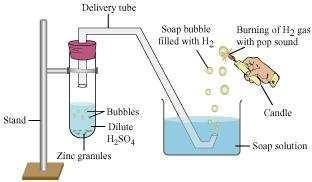Take few pieces of zinc granules and add 5 ml of dilute H2SO4. Shake it and pass the gas produced into a soap solution. The bubbles of the soap solution are formed. These soap bubbles contain hydrogen gas.

We can test the evolved hydrogen gas by its burning with a pop sound when a candle is brought near the soap bubbles.

Question 3:

Metal compound A reacts with dilute hydrochloric acid to produce effervescence. The gas evolved extinguishes a burning candle. Write a balanced chemical equation for the reaction if one of the compounds formed is calcium chloride.

Question 1:

Why do HCl, HNO3, etc., show acidic characters in aqueous solutions while solutions of compounds like alcohol and glucose do not show acidic character?

The dissociation of HCl or HNO3 to form hydrogen ions always occurs in the presence of water. Hydrogen ions (H+) combine with H2O to form hydronium ions (H3O+).

The reaction is as follows: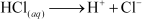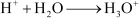Although aqueous solutions of glucose and alcohol contain hydrogen, these cannot dissociate in water to form hydrogen ions. Hence, they do not show acidic character.

Question 2:

Why does an aqueous solution of an acid conduct electricity?

Acids dissociate in aqueous solutions to form ions. These ions are responsible for conduction of electricity.

Question 3:

Why does dry HCl gas not change the colour of the dry litmus paper?

Colour of the litmus paper is changed by the hydrogen ions. Dry HCl gas does not contain H+ ions. It is only in the aqueous solution that an acid dissociates to give ions. Since in this case, neither HCl is in the aqueous form nor the litmus paper is wet, therefore, the colour of the litmus paper does not change.

Question 4:

While diluting an acid, why is it recommended that the acid should be added to water and not water to the acid?

Since the process of dissolving an acid in water is exothermic, it is always recommended that acid should be added to water. If it is done the other way, then it is possible that because of the large amount of heat generated, the mixture splashes out and causes burns.

Question 5:

How is the concentration of hydronium ions (H3O+) affected when a solution of an acid is diluted?

When an acid is diluted, the concentration of hydronium ions (H3O+) per unit volume decreases. This means that the strength of the acid decreases.

Question 6:

How is the concentration of hydroxide ions (OH) affected when excess base is dissolved in a solution of sodium hydroxide?

The concentration of hydroxide ions (OH) would increase when excess base is dissolved in a solution of sodium hydroxide.

Question 1:

You have two solutions, A and B. The pH of solution A is 6 and pH of solution B is 8. Which solution has more hydrogen ion concentration? Which of this is acidic and which one is basic?

A pH value of less than 7 indicates an acidic solution, while greater than 7 indicates a basic solution. Therefore, the solution with pH = 6 is acidic and has more hydrogen ion concentration than the solution of pH = 8 which is basic.

Question 2:

What effect does the concentration of ions have on the nature of the solution?

Concentration of can have a varied effect on  the nature of the solution. With an  increase in H+ ion concentration, the solution becomes more acidic, while a decrease of H+ ion causes an increase in the basicity of the solution.

Question 3:

Do basic solutions also have ions? If yes, then why are these basic?

Yes, basic solution also has ions. However, their concentration is less as compared to the concentration of OH ions that makes the solution basic.

Question 4:

Under what soil condition do you think a farmer would treat the soil of his fields with quick lime (calcium oxide) or slaked lime (calcium hydroxide) or chalk (calcium carbonate)?

If the soil is acidic and improper for cultivation, then to increase the basicity of soil, the farmer would treat the soil with quick lime or slaked lime or chalk.

Question 1:

What is the common name of the compound CaOCl2

The common name of the compound CaOCl2 is bleaching powder.

Question 2:

Name the substance which on treatment with chlorine yields bleaching powder?

Calcium hydroxide [Ca (OH)2], on treatment with chlorine, yields bleaching powder.

Question 3:

Name the sodium compound which is used for softening hard water.

Washing soda (Na2CO3.10H2O) is used for softening hard water.

Question 4:

What will happen if a solution of sodium hydrocarbonate is heated? Give the equation of the reaction involved.

When a solution of sodium hydrocarbonate (sodium hydrogencarbonate) is heated, sodium carbonate and water are formed with the evolution of carbon dioxide gas.Question 5:

Write an equation to show the reaction between Plaster of Paris and water.

The chemical equation for the reaction of Plaster of Paris and water can be represented as

Exercise solution

Question 1:

A solution turns red litmus blue, its pH is likely to be (a) 1 (b) 4 (c) 5 (d) 10

(d) Bases turn red litmus blue and acids turn blue litmus red. Basic solution has a pH value more than 7. Since the solution turns red litmus blue, its pH is likely to be 10.

Question 2:

A solution reacts with crushed egg-shells to give a gas that turns lime-water milky. The solution contains

1. NaCl (b) HCl (c) LiCl (d) KCl

1. The solution contains HCl.

Question 3:

10 mL of a solution of NaOH is found to be completely neutralised by 8 mL of a given solution of HCl. If we take 20 mL of the same solution of NaOH, the amount of HCl solution (the same solution as before) required to neutralise it will be

(a) 4 mL (b) 8mL (c) 12 mL (d) 16mL

(d) 16 mL of HCl solution will be required.

Question 4:

Which one of the following types of medicines is used for treating indigestion?

1. Antibiotic
2. Analgesic
3. Antacid
4. Antiseptic

(c) Antacid is used for treating indigestion.

Question 5:

Write word equations and then balanced equations for the reaction taking place when −

1. dilute sulphuric acid reacts with zinc granules.
2. dilute hydrochloric acid reacts with magnesium ribbon.
3. dilute sulphuric acid reacts with aluminium powder.
4. dilute hydrochloric acid reacts with iron filings.

1. Sulphuric acid + Zinc → Zinc sulphate + Hydrogen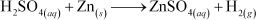1. Hydrochloric acid + Magnesium → Magnesium chloride + Hydrogen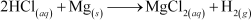1. Sulphuric acid + Aluminium → Aluminium sulphate + Hydrogen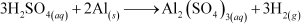1. Hydrochloric acid + Iron → Ferric chloride + HydrogenQuestion 6:

Compounds such as alcohols and glucose also contain hydrogen but are not categorized as acids. Describe an activity to prove it.

Two nails are fitted on a cork and are kept it in a 100 mL beaker. The nails are then connected to the two terminals of a 6-volt battery through a bulb and a switch. Some dilute HCl is poured in the beaker and the current is switched on. The same experiment is then performed with glucose solution and alcohol solution.

Observations:

It will be observed that the bulb glows in the HCl solution and does not glow in the glucose solution.

Result:

HCl dissociates into H+ and Cl ions. These ions conduct electricity in the solution resulting in the glowing of the bulb. On the other hand, the glucose solution does not dissociate into ions. Therefore, it does not conduct electricity.

Conclusion:

From this activity, it can be concluded that all acids contain hydrogen but not all compounds containing hydrogen are acids.

That is why, though alcohols and glucose contain hydrogen, they are not categorised as acids.

Question 7:

Why does distilled water not conduct electricity, whereas rain water does?

Distilled water is a pure form of water and is devoid of any ionic species. Therefore, it does not conduct electricity. Rain water, being an impure form of water, contains many ionic species such as acids and therefore it conducts electricity.

Question 8:

Why do acids not show acidic behaviour in the absence of water?

Acids do not show acidic behaviour in the absence of water because the dissociation of hydrogen ions from an acid occurs in the presence of water only. It is the hydrogen ions that are responsible for the acidic behaviour.

Question 9:

Five solutions A, B, C, D and E when tested with universal indicator showed pH as 4, 1, 11, 7 and 9, respectively. Which solution is

1. neutral?
2. strongly alkaline?
3. strongly acidic?
4. weakly acidic?
5. weakly alkaline?

Arrange the pH in increasing order of hydrogen-ion concentration.

1. Neutral → Solution D with pH 7
2. Strongly alkaline → Solution C with pH 11
3. Strongly acidic → Solution B with pH 1
4. Weakly acidic → Solution A with pH 4
5. Weakly alkaline → Solution E with pH 9

The pH can be arranged in the increasing order of the concentration of hydrogen ions as: 11 < 9 < 7 < 4 < 1

Question 10:

Equal lengths of magnesium ribbons are taken in test tubes A and B. Hydrochloric acid (HCl) is added to test tube A, while acetic acid (CH3COOH) is added to test tube B. In which test tube will the fizzing occur more vigorously and why?

The fizzing will occur strongly in test tube A, in which hydrochloric acid (HCl) is added. This is because HCl is a stronger acid than CH3COOH and therefore produces hydrogen gas at a faster speed due to which fizzing occurs.

Question 11:

Fresh milk has a pH of 6. How do you think the pH will change as it turns into curd? Explain your answer.

The pH of milk is 6. As it changes to curd, the pH will reduce because curd is acidic in nature. The acids present in it decrease the pH.

Question 12:

A milkman adds a very small amount of baking soda to fresh milk.

1. Why does he shift the pH of the fresh milk from 6 to slightly alkaline?
2. Why does this milk take a long time to set as curd?

1. The milkman shifts the pH of the fresh milk from 6 to slightly alkaline because in alkaline condition, milk does not set as curd easily.
2. Since this milk is slightly basic than usual milk, acids produced to set the curd are neutralized by the base. Therefore, it takes a longer time for the curd to set.

Question 13:

Plaster of Paris should be stored in a moisture-proof container. Explain why?

Plaster of Paris (POP) should be stored in a moisture-proof container because Plaster of Paris, a powdery mass, absorbs water (moisture) to form a hard solid known as gypsum.

Question 14:

What is a neutralization reaction? Give two examples.

A reaction in which an acid and base react with each other to give a salt and water is termed as neutralization reaction. In this reaction, energy is evolved in the form of heat. For example: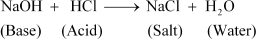(i)

(ii)  During indigestion (caused due to the production of excess of hydrochloric acid in the stomach), we administer an antacid (generally milk of   magnesia, which is basic in nature). The antacid neutralizes the excess of acids and thus gives relief from indigestion.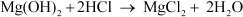Question 15:

Give two important uses of washing soda and baking soda.

Two important uses of washing soda and baking soda are as follows:

1. Washing soda:
1. It is used in glass, soap, and paper industries.
2. It is used to remove permanent hardness of water.
2. Baking soda:
1. It is used as baking powder. Baking powder is a mixture of baking soda and a mild acid known as tartaric acid. When it is heated or mixed in water, it releases CO2 that makes bread or cake fluffy.
2. It is used in soda-acid fire extinguishers.

NCERT Solutions for Class 10 Chapter 2 Acids, bases and salts are prepared to help students in their exam preparation. This solution provides you with answers to the questions provided in the NCERT Class 10 textbooks. To score better marks in Class 10 Science examination students should get well versed with the NCERT Solutions provided here.

In this chapter, students will learn about the nature and behaviour of Acids, bases and salts. This chapter mainly describes the chemical nature of acids, bases and salts, their reaction with metals, non-metals and with each other. This chapter is quite interesting as it involves many practical experiments to help you learn the basics.

Every year 3- 4 questions from this chapter are asked in Class 10 board examination. This is also one of the important chapters as the chances of scoring full marks on questions from this chapter are more. Hence, students are advised to practise as many questions from this chapter in order to solve every possible question that may be asked in the CBSE Class 10 Science examination.

Practical based multiple choice questions and subjective based questions are regularly asked in previous year question papers. Chapter 2 Acids, Bases and Salts

### Access Answers of Science NCERT class 10 Chapter 2 – Acids Bases and Salts

In-text questions set 1 —> Page number 18

1. You are given three test tubes. The three test tubes contain distilled water, acidic solution and the basic solution respectively. There is only red litmus paper available in order to identify what is there in each test tube. How will you find out what is in each of the test tubes?

Solution: We can identify the content in each of the test tubes using red litmus paper. This can be done by noticing the colour change of the red litmus paper.

• On litmus paper, the three solutions in the test tubes are poured separately.
• The solution which turns red litmus to blue contains a basic solution.
• Divide the formed blue litmus paper into two parts.
• The solution from the test tube which turns blue litmus paper to red will be the acidic solution.
• Solution of the test tube which do not change either red or blue litmus paper contain water.

NOTE: After immediate distillation, distilled water has a pH of 7. However, just within a few hours after distillation, it absorbs carbon dioxide from the atmosphere and turns slightly acidic with a pH of 5.8.

In-text questions set 2 —> Page number 22

1. Why should curd and sour substances not be kept in brass and copper vessels?

Solution: Curd and sour food substances contain acids; these acidic substances combine with metal. This reaction turns food to poison which damage people’s health.

2. Which gas is usually liberated when an acid reacts with a metal? Illustrate with an example. How will you test for the presence of this gas?

Solution: When an acid reacts with any metal, salt and hydrogen gas are formed.

Metal + Acid → Salt + Hydrogen gas

3. Metal compound A reacts with dilute hydrochloric acid to produce effervescence. The gas evolved extinguishes a burning candle. Write a balanced chemical equation for the reaction if one of the compounds formed is calcium chloride.

Solution: As metal compound released is Calcium Chloride the gas evolved here is CO2. Hence metal A should be Calcium Carbonate. Hence the reaction between Calcium Carbonate and HCl is

CaCO(s) + 2HCl (Aq) → CaCl2( Aq) + CO(g) + H2O (l)

in text questions set 3 Page number – 25

1. Why do HCl, HNO3, etc., show acidic characters in aqueous solutions while solutions of compounds like alcohol and glucose do not show acidic character?

Solution: Release of H+ ion in water will make a compound acidic or non-acidic. Acids are the substance which upon dissociating with water results in production of Hydrogen ions. Some compounds show acidic character as they dissociate in the aqueous solution which results in the production of hydrogen ions (acids like HCl, HNO3).

Compounds similar to glucose or alcohol do contain hydrogen element but they do not show signs of acidic nature. The fact that the hydrogen in them will not separate as like the hydrogen in the acids. They will not separate to become hydrogen ions, on dissolving in the water.

2. Why does an aqueous solution of an acid conduct electricity?

Solution: Charged particles are responsible for the conductance of electricity in an acid. These charged particles called as ions are the reason behind conductance of electricity in acid.

3. Why does dry HCl gas not change the colour of the dry litmus paper?

Solution: HCl does not give out Hydrogen ions, therefore HCl does not show any acidic behaviour and colour of the litmus paper remain the same on reacting with HCl gas.

4. While diluting an acid, why is it recommended that the acid should be added to water and not water to the acid?

Solution: While diluting an acid, it is recommended that the acid should be added to water and not water to the acid because if water is added to concentrated acid, it release huge amount of heat which may result in explosion and can cause acid burns on face, clothes and body parts. Hence it is safe to add acid to water but not water to acid.

5. How is the concentration of hydronium ions (H3O+) affected when a solution of an acid is diluted?

Solution: When acid is added to water there will be a fixed amount of hydronium present in the fixed volume of solution. If we dilute the solution hydronium ion per volume of solution decrease, this in-turn decreases Hydronium concentration in the solution.

6. How is the concentration of hydroxide ions (OH) affected when excess base is dissolved in a solution of sodium hydroxide?

Solution: When base is dissolved in sodium hydroxide solution its hydroxide ions increase but it will reach saturation at some point. After saturation point hydroxide ion concentration is not affected even after adding base further.

In-text questions set 4 Page number – 33

1. You have two solutions, A and B. The pH of solution A is 6 and pH of solution B is 8. Which solution has more hydrogen ion concentration? Which of this is acidic and which one is basic?

Solution: In order to find the hydrogen ion concentration, we can use the rule that states, “The pH of any solution is inversely proportional to the hydrogen ion concentration”. Therefore, it means that the solution that has a lower pH number will have a higher hydrogen ion concentration. Hence, solution A will have a higher hydrogen ion concentration. In addition, solution B will be basic and A will be acidic.

2. What effect does the concentration of H+(aq) ions have on the nature of the solution?

Solution: Hydrogen ion concentration decides the nature of the solution. If Hydrogen ion concentration increase then solution turn acidic and similarly if Hydrogen ion concentration decreases then solution turn basic.

3. Do basic solutions also have H+(aq) ions? If yes, then why are these basic?

Solution: Basic solutions has H+ ions, but hydroxide ions present in basic solution are more in basic solution. Hence Hydroxide ions turn solution to basic.

4. Under what soil condition do you think a farmer would treat the soil of his fields with quick lime (calcium oxide) or slaked lime (calcium hydroxide) or chalk (calcium carbonate)?

Solution: If the soil is acidic in nature (PH below 7) then such field should be treated with quick lime (calcium oxide) or slaked lime (calcium hydroxide) or chalk (calcium carbonate).

In text questions set 5 Page number – 34-35

1. What is the common name of the compound CaOCl2?

Solution: Common name of CaOCl2 is bleaching powder.

2. Name the substance which on treatment with chlorine yields bleaching powder

Solution: The substance which on treatment with chlorine yields bleaching powder is Calcium hydroxide.

3. Name the sodium compound which is used for softening hard water.

Solution: Sodium carbonate is the compound which is used for softening hard water.

4. What will happen if a solution of sodium hydrocarbonate is heated? Give the equation of the reaction involved.

Solution: Heating sodium hydrocarbonate yields sodium carbonate and carbon dioxide gas is liberated in the process.

5. Write an equation to show the reaction between Plaster of Paris and water.

Solution: The chemical equation for the reaction of Plaster of Paris and water is

CaSO4.1/2H2O + 3/2H2O → CaSO4.2H2O

Exercise questions Page number – 33

1. A solution turns red litmus blue, its pH is likely to be

a) 1 (b) 4 (c) 5 (d) 10

Solution: Answer is 10 because litmus paper turns blue when reacts with basic solution (PH more than 7). Hence 10 is the answer.

2. A solution reacts with crushed egg-shells to give a gas that turns lime-water milky. The solution contains

a) NaCl (b) HCl (c) LiCl (d) KCl

Egg shells contains calcium carbonate, which on reaction with HCl liberates CO2 gas which turn lime water to milky.

CaCO3 + 2HCl → CaCl2 + H2O + CO2

3. 10 mL of a solution of NaOH is found to be completely neutralised by 8 mL of a given solution of HCl. If we take 20 mL of the same solution of NaOH, the amount HCl solution (the same solution as before) required to neutralise it will be

(a) 4 mL (b) 8 mL (c) 12 mL (d) 16 mL

Solution: Since 10 ml of NaOH requires 8 mL of HCL, 20 ml of NaOH require 8 x 2 = 16mL of HCl. Hence the answer is option d 16mL.

4. Which one of the following types of medicines is used for treating indigestion?

(a) Antibiotic (b) Analgesic (c) Antacid (d) Antiseptic

Solution: Indigestion is due to excess production of acid in the stomach. Medicines used to treat indigestion is called as Antacid.

5. Write word equations and then balanced equations for the reaction taking place when

(a) Dilute sulphuric acid reacts with zinc granules.

(b) Dilute hydrochloric acid reacts with magnesium ribbon.

(c) Dilute sulphuric acid reacts with aluminium powder.

(d) Dilute hydrochloric acid reacts with iron filings.

Solution:

(a) dilute sulphuric acid reacts with zinc granules:

=> dilute sulphuric acid + zinc → Zinc Sulphate + Hydrogen Gas

=> H2SO4(aq) + Zn → ZnSO4(aq) + H2(g)

(b) dilute hydrochloric acid reacts with magnesium ribbon.

=> dilute Hydrochloric + Magnesium → Magnesium Chloride + Hydrogen Gas

=> 2HCl(aq) + Mg → MgCl2(aq) + H2(g)

(c) dilute sulphuric acid reacts with aluminium powder.

=> dilute Sulphuric Acid + Aluminium → Aluminium Sulphate + Hydrogen Gas

=> 3H2SO4(aq) + 2Al(s) → Al2(SO4)3(aq) + 3H2(g)

(d) dilute hydrochloric acid reacts with iron filings.

=> dilute Hydrochloric Acid + Iron → Ferrous Chloride + Hydrogen Gas

=> 6HCl(aq) + 3Fe(s) → 3FeCl2(aq) + 3H2(g)

6. Compounds such as alcohols and glucose also contain hydrogen but are not categorised as acids. Describe an Activity to prove it

Solution: Insert two nails on the wooden or rubber cork and place them on a beaker as shown in figure. Connect iron nail to a bulb, 6 volt battery and a wire connected to switch. Pour some alcohol or glucose so as to dip the nails in glucose or alcohol. Turn the switch on and you the see the bulb not glowing despite of connection to switch. Now empty the beaker and add HCL solution. This time bulb glows. This proves acid can conduct electricity but alcohol and glucose does not conduct electricity.

7. Why does distilled water not conduct electricity, whereas rain water does?

Solution:

• Distilled water does not contain any ionic compounds in it.
• Whereas rainwater has a lot, more compounds.
• Rainwater has dissolved acidic gas such as carbon dioxide from the air and that forms carbonic acid. This means that it has hydrogen ions and carbonate ions. Therefore, with the presence of acids, rainwater can conduct electricity.

8. Why do acids not show acidic behaviour in the absence of water?

Solution: The acidic behaviour from acids is because of the presence of hydrogen ions. Hydrogen ions can only be produced in the presence of water and therefore water is definitely needed if acids are to show their acidic behaviour.

9. Five solutions A, B, C, D and E when tested with universal indicator showed pH as 4, 1, 11, 7 and 9, respectively. Which solution is

(a) neutral?

(b) Strongly alkaline?

(c) Strongly acidic?

(d) Weakly acidic?

(e) Weakly alkaline?

Solution: In increasing order of hydrogen ion concentration:

pH 11(C) < pH 9(E) < pH 7 (D) <  pH 4 (A) < pH 1 (B)

PH11 – Strongly alkaline

pH9 – weakly alkaline

PH7 – Neutral

pH4 – Weakly acidic

pH1 – Strongly acidic

10. Equal lengths of magnesium ribbons are taken in test tubes A and B. Hydrochloric acid (HCl) is added to test tube A, while acetic acid (CH3COOH) is added to test tube B. Amount and concentration taken for both the acids are same. In which test tube will the fizzing occur more vigorously and why?

Solution: HCl is a strong acid whereas acetic is a weaker acid. Fizzing occurs because of the production of the hydrogen gas obtained due to reaction of the acid on the magnesium ribbon. Since HCl is a very strong acid there is a lot of liberation of hydrogen gas from test tube A. therefore, more fizzing take place in test tube A.

11. Fresh milk has a pH of 6. How do you think the pH will change as it turns into curd? Explain your answer.

Solution: Fresh milk is turned to curd due to production of lactic acid. Lactic acid reduces the pH of the milk.

12. A milkman adds a very small amount of baking soda to fresh milk.

(a) Why does he shift the pH of the fresh milk from 6 to slightly alkaline?

(b) Why does this milk take a long time to set as curd?

Solution: (a) He shifted the pH of the fresh milk from 6 to slightly alkaline to prevent milk from getting sour due to production of lactic acid.

(b) This milk takes long time to set into curd because the lactic acid produced here first neutralises the pH then the pH is reduced to turn milk to curd.

13. Plaster of Paris should be stored in a moisture-proof container. Explain why?

Solution: Plaster of Paris should be stored in moisture-proof container because moisture can affect plaster of Paris by slowing down the setting of the plaster because of hydration. This will turn plaster useless.

14. What is a neutralisation reaction? Give two examples.

Solution: The reaction of the acid + base gives a product of salt + water, which is considered as neutralization reaction.

Examples:

NaOH + HCl → NaCl + H2O

Mg(OH)+ H2CO3 → MgCO3 + 2H2O

15. Give two important uses of washing soda and baking soda.

Solution:

Topics involved in Chapter 2 – Acid, bases and salts

• Understanding the chemical properties of bases and acids
• How do acids and bases react with metals?
• How do metal carbonates and metal hydrogen carbonates react with acids?
• How do acids and bases react with each other?
• The reaction of metallic oxides with acids
• The reaction of non – metallic oxide with base
• What do all acids and bases have in common?
• What happens to an acid or base in a water solution?
• How strong are Acid or base solutions?
• Importance of pH in everyday life
• Family of salts
• pH of salts
• Chemicals from common salt

### Conclusion

The NCERT Solution for Class Xth Science Chapter 2 Acid, Base, and Salts by Swastik Classes is an excellent resource for students to learn about the fundamental concepts of acids, bases, and salts. The chapter provides a comprehensive understanding of the properties of acids and bases, pH scale, indicators, neutralization reactions, and the preparation and properties of different salts.
The Swastik Classes offer detailed explanations and examples to help students easily understand complex concepts. The solutions also provide practice questions and exercises to help students test their knowledge and understanding of the chapter.
Overall, this chapter is essential for students who wish to pursue a career in science or any related field. With the help of the NCERT Solution for Class Xth Science Chapter 2 Acid, Base, and Salts by Swastik Classes, students will be able to build a strong foundation in the field of chemistry and understand the role of acids, bases, and salts in our daily lives.

#### Features of NCERT Solutions for Class 10 Chapter 2 Science Acids bases and salts

• There are a lot of practical based questions in this Solution and these types of questions are often asked in CBSE Class 10 Science examination.
• You will encounter questions on a day to day examples of acid bases and salts.
• One can perceive different kinds of reactions involved with acids, bases and salts.

SWC is India’s top education technology company which believes in a modern learning approach. Our resource materials include NCERT notes, books, animations, videos, info-graphics, NCERT exemplar solutions, sample papers and NCERT Solutions. Our study materials will not only help you in understanding the topic completely but also help you in memorising the topic for a very long period of time.

## Frequently Asked Questions on NCERT Solutions for Class 10 Science Chapter 2

### List out the concepts discussed in the Chapter 2 of NCERT Solutions for Class 10 Science.

The concepts discussed in the Chapter 2 of NCERT Solutions for Class 10 Science are –
1. Understanding the chemical properties of bases and acids
2. How do acids and bases react with metals?
3. How do metal carbonates and metal hydrogen carbonates react with acids?
4. How do acids and bases react with each other?
5. The reaction of metallic oxides with acids
6. The reaction of non – metallic oxide with base
7. What do all acids and bases have in common?
8. What happens to an acid or base in a water solution?
9. How strong are Acid or base solutions?
10. Importance of pH in everyday life